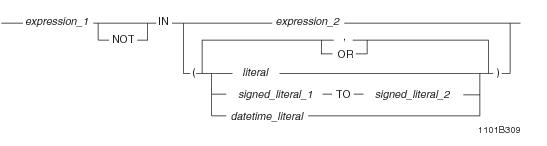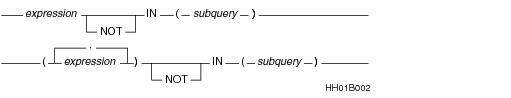# 15.00 - IN/NOT IN - Teradata Database

## Teradata Database SQL Functions, Operators, Expressions, and Predicates

Product
Release Number
15.00
Content Type
Programming Reference
Publication ID
B035-1145-015K
Language
English (United States)

## IN/NOT IN

### Purpose

Tests the existence of the value of an expression or expression list in a comparable set in one of two ways:

• Compares the value of an expression with values in an explicit list of literals.
• Compares values in a list of expressions with values and in a set of corresponding expressions in a subquery.
• ### ANSI Compliance

IN and NOT IN are ANSI SQL:2011 compliant.

Using TO in a list of literals is a Teradata extension to the ANSI standard.

### Syntax : expression IN and NOT IN expression or literalswhere:

 Syntax element … Specifies … expression_1 the value of the expression whose existence is to be tested in expression_2 or in an explicit list of literals named by literal, signed_literal TO signed_literal, or datetime_literal. IN NOT IN whether the test is inclusive or exclusive. You can substitute: IN ANY IN SOME = ANY = SOME for IN, unless a list of literals is specified and includes signed_literal_1 TO signed_literal_2 You can substitute: <> ALL NOT IN ALL for NOT IN, unless a list of literals is specified and includes signed_literal_1 TO signed_literal_2 expression_2 the value in which the existence of expression_1 is to be tested. literal literal macro parameter built-in value such as TIME or DATE signed_literal_1 TO signed_literal_2 a range of literals. datetime_literal an ANSI DateTime literal.

### Result

If IN is used with a list of literals, the result is true if the value of expression_1 is:

• equal to any literal in the list,
• between signed_literal_1 and signed_literal_2, inclusively, when signed_literal_1 is less than or equal to signed_literal_2, or
• between signed_literal_2 and signed_literal_1, inclusively, when signed_literal_2 is less than signed_literal_1
• If the value of expression_1 is null, then the result is considered to be unknown.

If the value of expression_1 is not null, and none of the conditions are satisfied for the result to be true, then the result is false.

Using this form, the IN search condition is satisfied if the expression is equal to any of the values in the list of literals; the NOT IN condition is satisfied if none of the values in the list of literals are equal to the expression.

 THE condition is true for this form … WHEN … expression_1 IN expression_2 expression_1 = expression_2 expression_1 NOT IN expression_2 expression_1 <> expression_2 expression_1 IN (const_1, const_2) (expression_1 = const_1) OR (expression_1 = const_2) expression_1 NOT IN (const_1, const_2) (expression_1 <> const_1) AND (expression_1 <> const_2) expression_1 IN (signed_const_1 TO signed_const_2) where signed_const_1 <= signed_const_2 (signed_const_1 <= expression_1) AND (expression_1 <= signed_const_2) expression_1 IN (signed_const_1 TO signed_const_2) where signed_const_2 < signed_const_1 (signed_const_2 <= expression_1) AND (expression_1 <= signed_const_1) expression_1 NOT IN (signed_const_1 TO signed_const_2) where signed_const_1 <= signed_const_2 (expression_1 < signed_const_1) OR (expression_1 > signed_const_2) expression_1 NOT IN (signed_const_1 TO signed_const_2) where signed_const_2 < signed_const_1 (expression_1 < signed_const_2) OR (expression_1 > signed_const_1)

Here are some examples:

 This statement … Is equivalent to this statement … `SELECT DeptNo FROM Department WHERE DeptNo IN (500, 600);` `SELECT DeptNo FROM DepartmentWHERE (DeptNo = 500) OR (DeptNo = 600); ` `UPDATE Employee SET Salary=Salary + 200 WHERE DeptNo NOT IN (100, 700);` `UPDATE Employee SET Salary=Salary + 200 WHERE (DeptNo ^= 100) AND (DeptNo ^= 700);`

### Usage Notes

If IN is used with a single‑term operator, that operator can be a literal or an expression. If a multiple-term operator is used, that operator must consist of literals; expressions are not allowed.

The expression_1 data type and the literal values must be compatible. Implicit conversion rules are the same as for the comparison operators.

### Relationship Between IN/NOT IN and EXISTS/NOT EXISTS

In general, you can use EXISTS to replace comparisons with IN, and NOT EXISTS to replace comparisons with NOT IN. However, the reverse is not true. The solutions to some problems require using the EXISTS or NOT EXISTS predicate. For information on EXISTS and NOT EXISTS, see “EXISTS / NOT EXISTS” on page 937.

### Equivalences Using IN/NOT IN, NOT, and ANY/ALL/SOME

The following table provides equivalences for the ANY/ALL/SOME quantifiers, where op is IN or NOT IN:

 This usage … Is equivalent to …1 `NOT (x op ALL (:a, :b, :c))` `x NOT op ANY (:a, :b, :c)` `x NOT op SOME (:a, :b, :c)` `NOT (x op ANY (:a, :b, :c))` `x NOT op ALL (:a, :b, :c)` `NOT (x op SOME (:a, :b, :c))` `NOT (x op (:a, :b, :c))` `x NOT op (:a, :b, :c)`

In the equivalences, if op is NOT IN, then NOT op is IN, not NOT NOT IN.

Here are some examples:

 This expression … Is equivalent to … `NOT (x IN ANY (:a, :b, :c))` `x NOT IN ALL (:a, :b, :c)` `NOT (x IN ALL (:a, :b, :c))` `x NOT IN ANY (:a, :b, :c)` `NOT (x NOT IN ANY (:a, :b, :c))` `x IN ALL (:a, :b, :c)` `NOT (x NOT IN ALL (:a, :b, :c))` `x IN ANY (:a, :b, :c)` `NOT (x IN (:a, :b, :c))` `x NOT IN (:a, :b, :c)` `NOT (x NOT IN (:a, :b, :c))` `x IN (:a, :b, :c)`

### Syntax 2: expression IN and NOT IN subquery

This syntax for IN and NOT IN is correct in either of the following two forms:where:

 Syntax element … Specifies … expression the value of the expression whose existence is to be tested in subquery. subquery a SELECT statement that returns values that satisfy the stated search criterion. The subquery must: Be enclosed in parentheses. Not end with a semicolon. Select the same number of expressions as are defined in the expression list. Not specify a SELECT AND CONSUME statement.

### Behavior of Nulls for IN

A statement result does not include column nulls when IN is used with a subquery.

### Behavior of Nulls for NOT IN

The following table explains the behavior of nulls for NOT IN for queries of various forms:

 FOR a query of the following form … IF … THEN … `SELECT ... FROM T1 WHERE x NOT IN (SELECT y FROM T2);` one of the y values is null no T1 rows are returned for the entire query. some rows are returned by the subquery, and if x contains some nulls those T1 rows that contain a null in x are not returned. `SELECT ... FROM T1 WHERE expression_list_1 NOT IN (SELECT expression_list_2   FROM T2);` a null is the first field in expression_list_2 no rows from T1 are returned. a null is in a field other than the first field of expression_list_2 some rows may be returned the subquery returns some rows, and if a null is in the first field in expression_list_1 the T1 rows containing a null in the first field of expression_list_1 are not returned. `SELECT ... FROM T1 WHERE expression_list_1 NOT IN (SELECT expression_list_2   FROM T2   WHERE search_condition);` the search_condition on T2 returns no rows all T1 rows, including those containing a NULL in the first field of expression_list_1, are returned.

### NOT IN Clauses and Stored Procedures

You cannot specify a NOT IN clause in a stored procedure conditional expression if that expression also references an alias for a local variable, parameter, or cursor.

### NOT IN and Recursive Queries

NOT IN cannot appear in a recursive statement of a recursive query. However, a non-recursive seed statement in a recursive query can specify the NOT IN predicate.

### Queries With Large NOT IN Clauses Can Fail

Queries that contain thousands of arguments within an IN or NOT IN clause sometimes fail.

For example, suppose you ran the following query with 16000 IN clause arguments, and it failed.

`   SELECT MAX(emp_num)`
`   FROM employee`
`   WHERE emp_num IN(1,2,7,8,...,121347);`

A workaround when this problem occurs is to rewrite the query using a temporary or volatile table to contain the arguments within the IN clause.

The following statements allow you to make the same selection, but without failure.

`   CREATE VOLATILE TABLE temp_IN_values (`
`    in_value INTEGER) ON COMMIT PRESERVE ROWS;`
`   `
`   INSERT INTO temp_IN_values`
`   SELECT emp_num`
`   FROM table_with_emp_num_values;`

The new query is as follows:

`   SELECT MAX(emp_num)`
`   FROM employee AS e JOIN temp_IN_values AS en`
`   ON (e.emp_num = en.in_value);`

### Example

The following statement searches for the names of all employees who work in Atlanta.

`   SELECT Name `
`   FROM Employee `
`   WHERE DeptNo IN`
`    (SELECT DeptNo `
`     FROM Department `
`     WHERE Loc = 'ATL');`

### Example

Using a similar example but assuming that the DeptNo is divided into two columns, the following statement could be used:

`   SELECT Name `
`   FROM Employee `
`   WHERE (DeptNoA, DeptNoB) IN`
`    (SELECT DeptNoA, DeptNoB `
`     FROM Department `
`     WHERE Loc = 'LAX') ;`

### Example

This example shows the behavior of IN/NOT IN with a list of literals.

Consider the following table definition and contents:

`   CREATE TABLE t (x INTEGER);`
`   INSERT t (1);`
`   INSERT t (2);`
`   INSERT t (3);`
`   INSERT t (4);`
`   INSERT t (5);`

 IF you use this query … THEN the result is … `SELECT * FROM t WHERE x IN (1,2)` `1, 2` `SELECT * FROM t WHERE x IN ANY (1,2)` `1, 2` `SELECT * FROM t WHERE NOT (x NOT IN (1,2))` `1, 2` `SELECT * FROM t WHERE x NOT IN (1,2)` `3, 4, 5` `SELECT * FROM t WHERE x NOT IN ALL (1,2)` `3, 4, 5` `SELECT * FROM t WHERE NOT (x IN (1, 2))` `3, 4, 5` `SELECT * FROM t WHERE NOT (x IN ANY (1,2))` `3, 4, 5` `SELECT * FROM t WHERE x IN (3 TO 5)` `3, 4, 5` `SELECT * FROM t WHERE x NOT IN SOME (1, 2)` `1, 2, 3, 4, 5` `SELECT * FROM t WHERE x IN (1, 2 TO 4, 5)` `1, 2, 3, 4, 5` `SELECT * FROM t WHERE x IN ALL (1,2)` no rows `SELECT * FROM t WHERE NOT (x NOT IN SOME (1,2))` no rows `SELECT * FROM t WHERE x NOT IN (1 TO 5)` no rows